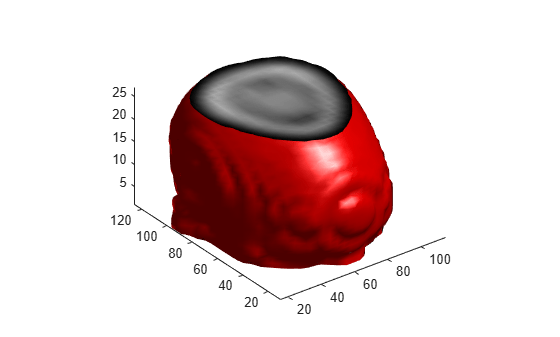# reducevolume

Reduce number of elements in volume data set

## Syntax

```[nx,ny,nz,nv] = reducevolume(X,Y,Z,V,[Rx,Ry,Rz]) [nx,ny,nz,nv] = reducevolume(V,[Rx,Ry,Rz]) nv = reducevolume(...) ```

## Description

`[nx,ny,nz,nv] = reducevolume(X,Y,Z,V,[Rx,Ry,Rz]) ` reduces the number of elements in the volume by retaining every `Rx`th element in the x direction, every `Ry`th element in the y direction, and every `Rz`th element in the z direction. If a scalar `R` is used to indicate the amount or reduction instead of a three-element vector, the MATLAB® software assumes the reduction to be ```[R R R]```.

The arrays `X`, `Y`, and `Z` define the coordinates for the volume `V`. The reduced volume is returned in `nv`, and the coordinates of the reduced volume are returned in `nx`, `ny`, and `nz`.

`[nx,ny,nz,nv] = reducevolume(V,[Rx,Ry,Rz]) ` assumes the arrays `X`, `Y`, and `Z` are defined as `[X,Y,Z] = meshgrid(1:n,1:m,1:p)`, where ```[m,n,p] = size(V)```.

`nv = reducevolume(...) ` returns only the reduced volume.

## Examples

collapse all

This example uses a data set that is a collection of MRI slices of a human skull. This data is processed in a variety of ways:

• The 4-D array is squeezed (`squeeze`) into three dimensions and then reduced (`reducevolume`) so that what remains is every fourth element in the x and y directions and every element in the z direction.

• The reduced data is smoothed (`smooth3`).

• The outline of the skull is an isosurface generated as a patch (`p1`) whose vertex normals are recalculated to improve the appearance when lighting is applied (`patch`, `isosurface`, `isonormals`).

• A second patch (`p2`) with an interpolated face color draws the end caps (`FaceColor`) isocaps).

• The view of the object is set (`view`, `axis`, `daspect`).

• A 100-element grayscale colormap provides coloring for the end caps (`colormap`).

• Adding a light to the right of the camera illuminates the object (`camlight`, `lighting`).

```load mri D = squeeze(D); [x,y,z,D] = reducevolume(D,[4,4,1]); D = smooth3(D); p1 = patch(isosurface(x,y,z,D,5),... 'FaceColor','red','EdgeColor','none'); isonormals(x,y,z,D,p1) p2 = patch(isocaps(x,y,z,D,5),... 'FaceColor','interp','EdgeColor','none'); view(3) axis tight daspect([1,1,.4]) colormap(gray(100)) camlight lighting gouraud```## Version History

Introduced before R2006a